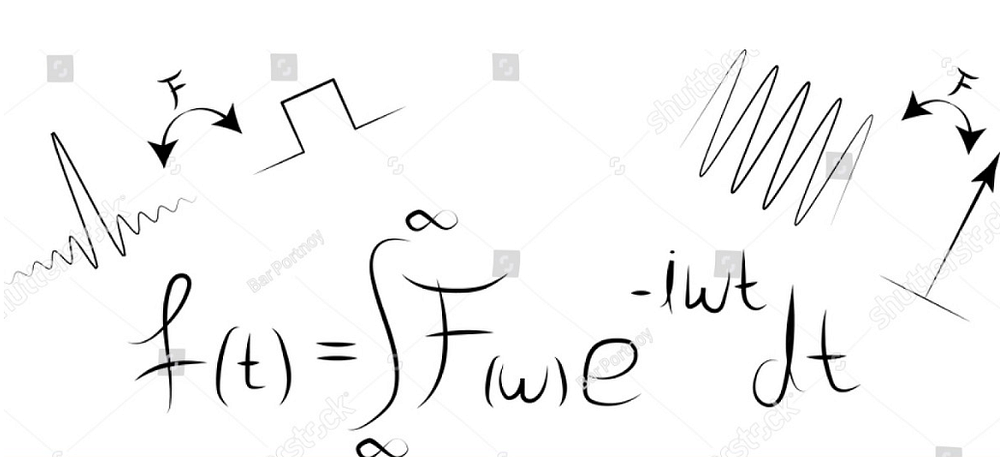# Fourier Transformation for a Data Scientist

Source: Deep Learning on MediumAlso, when we actually solve the above integral, we get these complex numbers where `a` and `b` correspond to the coefficients that we are after.

The continuous Fourier transform converts a time-domain signal of infinite duration into a continuous spectrum composed of an infinite number of sinusoids. In practice, we deal with signals that are discretely sampled, usually at constant intervals, and of finite duration or periodic. For this purpose, the classical Fourier transform algorithm can be expressed as a Discrete Fourier transform (DFT), which converts a finite sequence of equally-spaced samples of a function into a same-length sequence of equally-spaced samples of the discrete-time Fourier transform: